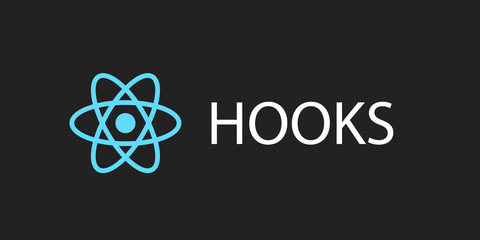## 回顾

1. 调用setState，就会触发组件的重新渲染，无论前后的state是否不同
2. 父组件更新，子组件也会自动的更新

## 对比

function useMemo<T>(factory: () => T, deps: DependencyList | undefined): T; function useCallback<T extends (...args: any[]) => any>(callback: T, deps: DependencyList): T;

useCallback和useMemo的参数跟useEffect一致，他们之间最大的区别有是useEffect会用于处理副作用，而前两个hooks不能。

useMemo和useCallback都会在组件第一次渲染的时候执行，之后会在其依赖的变量发生改变时再次执行；并且这两个hooks都返回缓存的值，useMemo返回缓存的变量，useCallback返回缓存的函数。

## useMemo

``````import React from 'react';

export default function WithoutMemo() {
const [count, setCount] = useState(1);
const [val, setValue] = useState('');

function expensive() {
console.log('compute');
let sum = 0;
for (let i = 0; i < count * 100; i++) {
sum += i;
}
return sum;
}

return <div>
<h4>{count}-{val}-{expensive()}</h4>
<div>
<button onClick={() => setCount(count + 1)}>+c1</button>
<input value={val} onChange={event => setValue(event.target.value)}/>
</div>
</div>;
}
``````

``````export default function WithMemo() {
const [count, setCount] = useState(1);
const [val, setValue] = useState('');
const expensive = useMemo(() => {
console.log('compute');
let sum = 0;
for (let i = 0; i < count * 100; i++) {
sum += i;
}
return sum;
}, [count]);

return <div>
<h4>{count}-{expensive}</h4>
{val}
<div>
<button onClick={() => setCount(count + 1)}>+c1</button>
<input value={val} onChange={event => setValue(event.target.value)}/>
</div>
</div>;
}
``````

## useCallback

const fnA = useCallback(fnB, [a])

``````import React, { useState, useCallback } from 'react';

const set = new Set();

export default function Callback() {
const [count, setCount] = useState(1);
const [val, setVal] = useState('');

const callback = useCallback(() => {
console.log(count);
}, [count]);

return <div>
<h4>{count}</h4>
<h4>{set.size}</h4>
<div>
<button onClick={() => setCount(count + 1)}>+</button>
<input value={val} onChange={event => setVal(event.target.value)}/>
</div>
</div>;
}
``````

``````import React, { useState, useCallback, useEffect } from 'react';
function Parent() {
const [count, setCount] = useState(1);
const [val, setVal] = useState('');

const callback = useCallback(() => {
return count;
}, [count]);
return <div>
<h4>{count}</h4>
<Child callback={callback}/>
<div>
<button onClick={() => setCount(count + 1)}>+</button>
<input value={val} onChange={event => setVal(event.target.value)}/>
</div>
</div>;
}

function Child({ callback }) {
const [count, setCount] = useState(() => callback());
useEffect(() => {
setCount(callback());
}, [callback]);
return <div>
{count}
</div>
}
``````

## 多谈一点

useEffect、useMemo、useCallback都是自带闭包的。也就是说，每一次组件的渲染，其都会捕获当前组件函数上下文中的状态(state, props)，所以每一次这三种hooks的执行，反映的也都是当前的状态，你无法使用它们来捕获上一次的状态。对于这种情况，我们应该使用ref来访问。

https://github.com/anymost/hooks-demo/blob/master/src/page/Memo.jsgithub.com
https://github.com/anymost/hooks-demo/blob/master/src/page/Callback.jsgithub.com

Richard：useEffect使用指南zhuanlan.zhihu.com